## Gravity

10-6-99

Sections 5.6 - 5.10

### The force of gravity

Isaac Newton is probably best known for his study of gravity, seeing as just about everyone has heard the story about Newton being conked on the head by an apple. What Newton said was this: whenever there are two objects that have mass, they will exert a gravitational force on each other that is proportional to the product of the masses, and inversely proportional to the square of the distance between them. (Actually, inversely proportional to the square of the distance between the two centers of mass is more accurate.) If the first object has a mass of m1, and the second object has a mass m2, and there is a distance r between them (between their centers of mass, that is), the magnitude of the gravitational force is given by: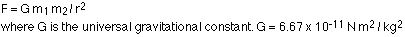That equation is Newton's universal law of gravitation.

You might be used to thinking of the gravitational force as F = mg. Where does this other, more complicated, equation fit in? F = mg is actually a special-case form of the other one, applying only to objects very close to the surface of the Earth. This value of g, 9.8 m/s2, can be found by combining G, the mass of the Earth, and the radius of the Earth. If you set F = mg equal to the gravitational force equation, you get: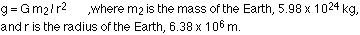Plugging these numbers in, and the value of G, gives g = 9.80 m/s2.

Any two masses exert equal-and-opposite gravitational forces on each other. If we drop a ball, the Earth exerts a gravitational force on the ball, but the ball exerts a gravitational force of the same magnitude (and in the opposite direction) on the Earth. The force just makes a lot less difference to the Earth because of its large mass.

Example - The Earth orbiting the Sun. What is the magnitude of the acceleration experienced by the Earth in its orbit around the Sun? We can work out the answer two different ways.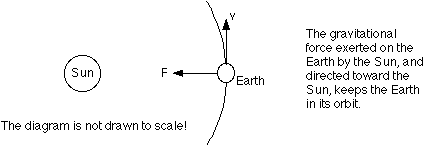The free-body diagram of the Earth in this situation involves only one force, the gravitational force exerted by the Sun on the Earth, given by Newton's law of universal gravitation, which we've seen before:The two masses are the mass of the Sun and the mass of the Earth, and r is the distance between them. Because this is the only force acting on the Earth, we can set this equal to ma, remembering that a has the special form a = v2 / r: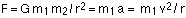This gives us two ways to get the acceleration,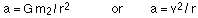Method 1 - To get the acceleration using the first equation, we need to know the mass of the Sun and the distance from the Earth to the Sun. Roughly, the Sun has a mass of 2 x 1030 kg, and it's 93 million miles = 150 million km = 1.5 x 1011 m away. Plugging these values in gives an acceleration of :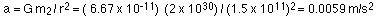Method 2 - the second method should give us the same answer, but we need to know the speed of the Earth as it orbits the Sun. The period, T, the time it takes the Earth to orbit the Sun, is one year, which, very conveniently, happens to work out to very close to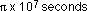. The speed of the Earth is: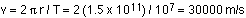This gives an acceleration of: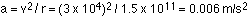That's pretty good agreement between the two methods, given the approximating we're doing here, which means the approximations are rather good. The acceleration is relatively small, but sufficient to keep the Earth in its orbit.

### Kepler's laws

Johannes Kepler (1571-1630) was a German astronomer who made some observations about the motion of the planets. He summarized those in three statements known as Kepler's laws.

The one that's perhaps the most relevant for our purposes is Kepler's third law, which states that the ratio of the squares of the periods of any two planets orbiting the Sun is equal to the ratio of the cubes of their average distances from the Sun.

Newton showed that Kepler's third law can be derived from Newton's law of universal gravitation. We've already figured out that applying Newton's second law results in :

G m1 m2 / r2 = m1 v2 / r

This simplifies to:

G m2 / r = v2 (where m2 represents the mass of the Sun)

If the orbit is circular, then the speed is simply the circumference of the orbit divided by the period, T, the time to travel once around the orbit.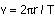Combining these two equations and eliminating v gives: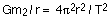This can be rearranged to get:This represents Kepler's third law. For every planet in the solar system, the right-hand side of the equation is the same, because it has only the universal gravitational constant and the mass of the Sun. Therefore the ratio of the square of the period to the cube of the average distance is the same value for every planet in the solar system.